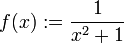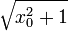# Locally analytic not implies globally analytic

It is possible to have a function$f$ defined on all of$\R$ such that$f$ is a locally analytic function everywhere on$\R$ but$f$ is not a globally analytic function.$f(x) := \frac{1}{x^2 + 1}$
This is locally analytic everywhere, but not globally analytic. In fact, the radius of convergence of its power series at any point$x_0$ is$\sqrt{x_0^2 + 1}$.